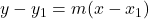Question

Write the Point Slope form for the line that has a slope of 4/3 and goes through the point 0, -3​

1.Answer:  y + 3 = 4/3 x

Step-by-step explanation:

Point Slope form is that, where m is the slope, and x1,y1 is the point.

We plug 4/3 for the slope m, and 0 for x1, -3 for y1, then we get:

y -(-3) = 4/3 (x-0)

y + 3 = 4/3 x